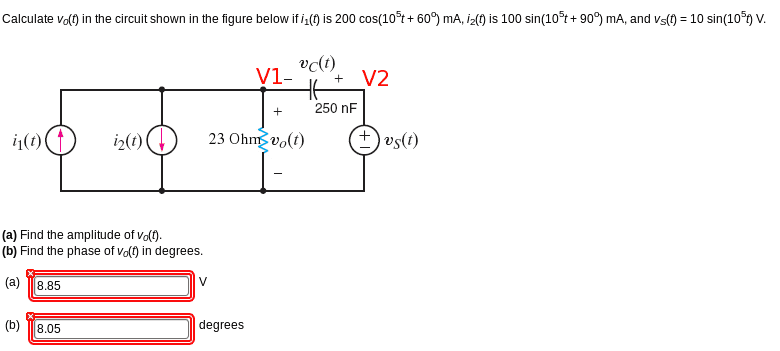# Phasor circuit analysis

• Engineering
• x86
In summary, the conversation discusses using KCL (Kirchoffs Current Law) and Z for the capacitor to solve a circuit problem. The attempt at a solution involves using a reference point and converting all current and voltage functions to phasors. After some corrections, the correct solution is found to be V1 = -6.04 - 0.51J. The conversation concludes with gratitude for the help and a reminder to be more careful in calculations.

## Homework Statement(Note, my answer below is also wrong. I've attempted this problem for about 1 hour now and I can't figure it out.)

## Homework Equations

KCL (Kirchoffs Current Law)
Z for the capacitor, Z = 1/(jwC)[/B]

## The Attempt at a Solution

I'm using KCL at V1 with the following convention: +ve for current flowing out of the node, -ve for current flowing in

If the bottom of our circuit is the reference:
V2 = Vs

KCL at Node 1
-i1(t) + i2(t) + V1/23 - (Vs-V1/(-40j) = 0

Now I convert all the current and voltage functions to phasors.
i1(t) = 0.2(60d)
i2(t) = 0.1(-90d)
Vs(t) = 10(-180d)

Subbing into above...

-0.2(60d) + 0.1(-90d) + V1/23 - (10(-180d))/(-40j) + V1/(-40j) = 0

V1( 1/23 + 1/(-40j) ) = 0.2(60d) - 0.1(-90d) + 10(-180d) / (-40j)

V1 = (0.1 + 0.1732j + 0.1j - 10/(40j)) / (1/23 + 1/(-40j))

V1 = 6.93 + 8.05j

Converting this to phasor form,

V=10.622(40.3d)

But this is also wrong. I can't figure out what I'm doing wrong.

Last edited:
x86 said:
i2(t) = 0.1(-90d)
Vs(t) = 10(-180d)

It looks like you are double-converting the signs. Do you really want to invert those phase angles? (It's been a long time since I used phasors, so maybe there is a reason).

•x86
berkeman said:
It looks like you are double-converting the signs. Do you really want to invert those phase angles? (It's been a long time since I used phasors, so maybe there is a reason).

Right, I think you are right. cosx = sin(x+90)

I'm going to try again with i2(t) = 0.1(0180d) = 0.1(0d) and Vs(t) = 10(90d)

-i1(t) + i2(t) + V1/23 - (Vs-V1/(-40j) = 0
-0.1(0d) + 0.2(60d) + V1/23 - 10(90d)/(-40j) + V1/(-40j) = 0

V1(1/23 + 1/(-40j)) = 0.1(0d) - 0.2(60d) + 10(90d) / (-40j)

V1 = -6.04 - 0.51 J

Yep. It is correct. Thank you, I really need to be more careful when calculating the little things :)

•berkeman